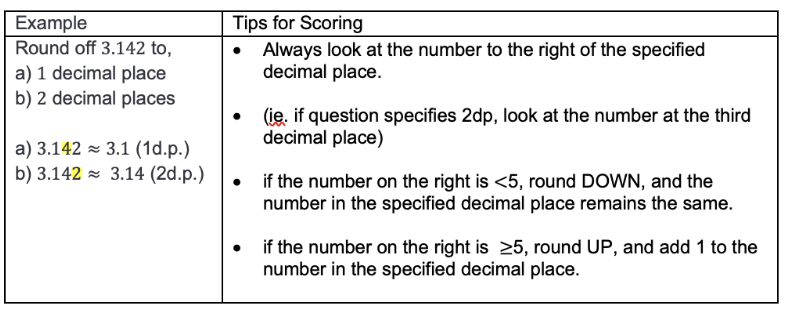top of page
Search

# 5 Common Questions on Secondary Math's 'Approximation and Estimation' Topic and How To Score ThemFor many students, the 'Approximation and Estimation' topic in Secondary Mathematics can often seem daunting. However, with the right approach, these essential skills can be mastered.

In this guide, we will break down the topic into five commonly asked questions to provide you and your child with a deeper understanding of different examples of approximation and estimation. Our aim is to help your child achieve higher scores and performance in this critical area and foster a lasting appreciation of the intrinsic beauty of mathematics.

## 1. Rounding to Decimal Places

Rounding to decimal places is a common mathematical concept, particularly in 'Approximation and Estimation'. It is a method used to simplify numbers while keeping their value close to the original.

When you round a number to a specific number of decimal places, you limit the digits that appear after the decimal point in a number. The process involves examining the digit in the position to the right of the desired decimal place and deciding whether to round up or down.

Below are tips for scoring:## 2. ​Rounding to Significant Figures

Rounding to significant figures is another method of approximation that is particularly useful in scientific and engineering calculations where precision is crucial. However, an exact number may not be required or possible.

Significant figures in a number are digits that provide meaningful information about its precision. This typically includes all digits except:

• Trailing zeros when they are merely placeholders to indicate the scale of the number.

Remember, rounding to significant figures aims to retain the necessary precision for a specific calculation or comparison without exceeding it. This helps minimise potential errors or misunderstandings arising from overly precise calculations.

Below are tips for scoring:## 3. Evaluating and Rounding with the Use of a Calculator

Evaluating and rounding using a calculator is a vital mathematical skill, combining precise computations and appropriate rounding. The process begins by inputting an equation into the calculator while adhering to mathematical hierarchy rules like BODMAS/BIDMAS.

It is important to ensure that the calculator's mode matches the mathematical context, such as using degree mode for trigonometry. The output often results in a long decimal, especially when division or complex calculations are involved.

The next step is determining the level of precision, which requires understanding whether to round to a specific decimal place or significant figures. The rounding process involves examining the digit immediately to the right of the chosen place value. If it is 5 or greater, the number is rounded up; if it is less than 5, the number is rounded down. Some calculators even have built-in functions for rounding.

Below are tips for scoring:## 4. Estimating Values Using Approximated Values

Estimating values using approximated values is a strategy for simplifying calculations, particularly when an exact answer is not necessary. This involves replacing each number in the calculation with a rounded or more convenient value, allowing for faster and easier computations. The level of approximation depends on the required accuracy.

You perform calculations using these approximated numbers, resulting in an estimate rather than the exact answer. For example, when multiplying 98 by 51, you might round them to the nearest ten or nearest 100, respectively.

Multiplying these approximated values gives 5000, which serves as an estimate for the original multiplication.

Remember, while this method efficiently provides approximate answers, the estimates may deviate from the actual result, especially if the numbers were significantly rounded. Therefore, it is best used in situations where precision is not critical.

Below are tips for scoring:## 5. Finding the Lower and Upper Limits of a Rounded Number

Finding the lower and upper limits of a rounded number provides a range within which the original, unrounded number is likely to exist. This method is useful when working with measurements or quantities that have been rounded, when you want to determine the potential range of the original values.

Below are tips for scoring:Mastering the 'Approximation and Estimation' section of Secondary Mathematics can be simplified by understanding these five core concepts: Rounding to Decimal Places, Rounding to Significant Figures,

Evaluating and Rounding with the Use of a Calculator, Estimating Values Using Approximated Values, and Finding the Lower and Upper Limits of a Rounded Number. Each of these techniques serves a unique purpose and can significantly help in obtaining accurate answers and grasping the intrinsic nature of mathematical problems.

Remember, the goal of estimate and approximate is not just about achieving numerical accuracy but also about fostering an appreciation for the beauty and logic of mathematics. These skills are essential for academic success and form the foundation of logical thinking and problem-solving in real-world situations.

With the proper understanding and practice, your child can confidently navigate these mathematical concepts, establishing a solid groundwork that will benefit them beyond their school years.

Let's ensure our children's success and improve their grades together. AGrader Learning Centre is composed of a team of experts dedicated to your child's academic growth.AGrader Secondary Math Tuition has been meticulously designed to align with the latest Ministry of Education standards, providing your child with a clear path to mastering mathematics. We offer a distinctive learning experience through our high-quality study materials and worksheets, carefully crafted to reflect the actual exam pattern. This familiarises students with the structure and builds confidence in their abilities.

Whether your child is preparing for the O Levels or is in Sec 1 to Sec 3, our committed and knowledgeable teachers are prepared to provide the support your child needs to conquer math challenges with ease.

Don't wait! Enrol your child in AGrader Learning Centre Secondary Math Tuition today and let them embark on a journey to success.